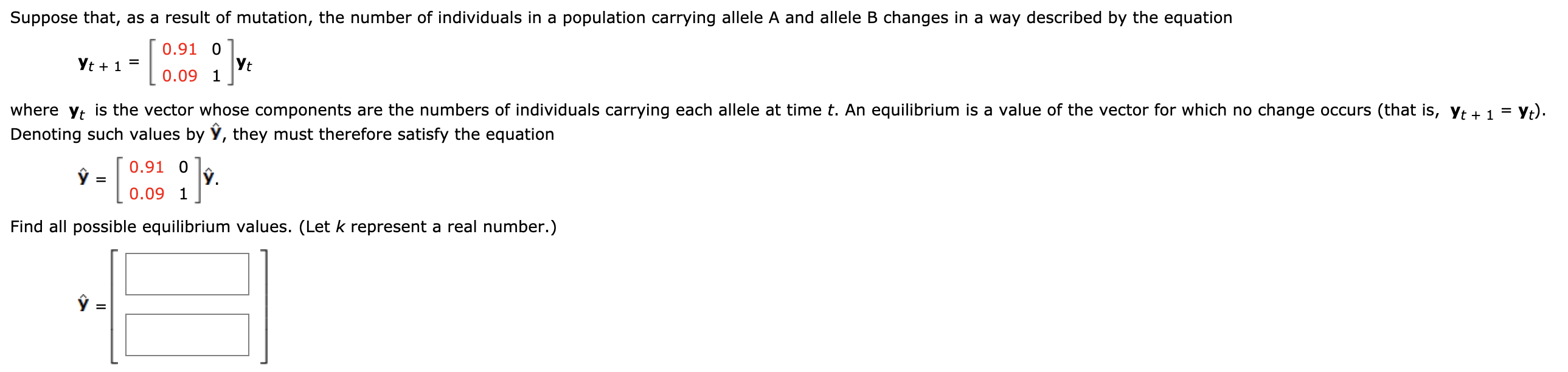# Question Solved1 AnswerSuppose that, as a result of mutation, the number of individuals in a population carrying allele A and allele B changes in a way described by the equation [ 0.91 01 Yt + 1 = 0.09 1] where yt is the vector whose components are the numbers of individuals carrying each allele at time t. An equilibrium is a value of the vector for which no change occurs (that is, Yt + 1 = yt). Denoting such values by ŷ, they must therefore satisfy the equation [ 0.91 Olo L 0.09 1] Find all possible equilibrium values. (Let k represent a real number.) y =Transcribed Image Text: Suppose that, as a result of mutation, the number of individuals in a population carrying allele A and allele B changes in a way described by the equation [ 0.91 01 Yt + 1 = 0.09 1] where yt is the vector whose components are the numbers of individuals carrying each allele at time t. An equilibrium is a value of the vector for which no change occurs (that is, Yt + 1 = yt). Denoting such values by ŷ, they must therefore satisfy the equation [ 0.91 Olo L 0.09 1] Find all possible equilibrium values. (Let k represent a real number.) y =
More
Transcribed Image Text: Suppose that, as a result of mutation, the number of individuals in a population carrying allele A and allele B changes in a way described by the equation [ 0.91 01 Yt + 1 = 0.09 1] where yt is the vector whose components are the numbers of individuals carrying each allele at time t. An equilibrium is a value of the vector for which no change occurs (that is, Yt + 1 = yt). Denoting such values by ŷ, they must therefore satisfy the equation [ 0.91 Olo L 0.09 1] Find all possible equilibrium values. (Let k represent a real number.) y =